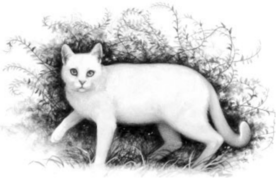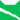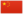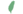—— 白翅，族群的战争》，第129页

Whitewing《族群的战争》雷族 武士雷族 猫后小白 Whitekit  小白
 白爪 Whitepaw  白掌
 白翅 Whitewing  白翅

Q2438：雷族母猫，自《午夜追踪》登场的角色

## 细节

### 外貌

• 她是一只身形娇小、:5章肩膀宽阔、:289皮毛柔软、:153绿色眼睛:猫物表的雪:120白色母猫。:猫物表

### 勘误

• 尽管在《日落和平》中提到她因怀孕而大腹便便，但在猫物表中她依然被列为武士。:猫物表虽然也可能是因为猫后经常持续执行武士职务到最后一刻为止。 :239
• 她曾被错误地称呼为“白尾”。:14
• 她曾被错误地描述为浅蓝色眼睛。:2章

## 登场书目

• 午夜追踪 (Q139)[配角][首次登场🐱]
• 新月危机 (Q140)[配角]
• 重现家园 (Q141)[配角]
• 星光指路 (Q142)[配角]
• 黄昏战争 (Q143)[配角]
• 日落和平 (Q144)[配角]
• 预视力量 (Q152)[配角]
• 暗河汹涌 (Q153)[配角]
• 驱逐之战 (Q154)[配角]
• 天蚀遮月 (Q155)[配角]
• 暗夜长影 (Q156)[配角]
• 拂晓之光 (Q157)[配角]
• 第四学徒 (Q158)[配角]
• 战声渐近 (Q159)[配角]
• 暗夜密语 (Q160)[配角]
• 月光印记 (Q161)[配角]
• 武士归来 (Q162)[配角]
• 群星之战 (Q163)[配角]
• 学徒探索 (Q170)[配角]
• 雷影交加 (Q171)[配角]
• 天空破碎 (Q172)[配角]
• 极夜无光 (Q173)[配角]
• 烈焰焚河 (Q174)[配角]
• 风暴来袭 (Q175)[配角]
• 迷失群星 (Q3563)[配角]
• 静默冰融 (Q3579)[猫物闲角]
• 叠影重障 (Q3624)[配角]
• 暗由心生 (Q4183)[配角]
• 无星之地 (Q4385)[配角]
• 迷雾明光 (Q4621)[猫物闲角]
• 河翻浪涌 (Q4643)[配角]
• 天穹将倾 (Q4799)[猫物闲角]
• 火星的探索 (Q176)[配角]
• 黑莓星的风暴 (Q182)[配角]
• 虎心的阴影 (Q185)[猫物闲角]
• 鸦羽的拷问 (Q186)[对话提及]
• 松鼠飞的希冀 (Q3561)[配角]
• 灰条的誓言 (Q4057)[猫物闲角]
• 冬青叶的故事 (Q187)[猫物闲角]
• 雾星的征兆 (Q188)[猫物闲角]
• 叶池的祈愿 (Q191)[配角]
• 鸽翅的沉默 (Q192)[配角]
• 褐皮的族群 (Q3566)[猫物闲角]
• 蛾翅的秘密 (Q3628)[猫物闲角]
• 黛西的至亲 (Q3629)[仅于幻象]
• 斑毛的反抗 (Q4337)[猫物闲角]
• 族群救星 (Q214)[配角]
• 族群的战争 (Q202)[配角]
• 终极指南 (Q204)[对话提及]

## 参考文献

这篇文章基于CC BY-SA 3.0许可使用了猫武士维基（英语）Whitewing一文中的部分内容。
1. 《火星的探索》尾声 (Q1691)
2. 午夜追踪 (Q139)预视力量 (Q152)
3. 预视力量 (Q152)
4. 鸦羽的拷问 (Q186)黑莓星的风暴 (Q182)
5. 黑莓星的风暴 (Q182)学徒探索 (Q170)
6. 叶池的祈愿
7. 群星之战
8. 午夜追踪
9. 重现家园
10. Kate Cary (2019-01-16). 站内截图. BlogClan . 访问于 2022-05-25. “Her purr is surprisingly loud. (...) Very occasionally, she purrs in her sleep.”
11. 寒冰烈火
12. 火星的探索
13. 拂晓之光
14. 钩星的承诺
15. 星光指路
16. 鸽翅的沉默